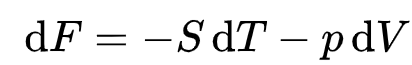# Fall 2023 Statistical Mechanics

Post statistical mechanics questions here

Why does the Helmholtz free energy has a “dT” (which seems to indicate it is one of its independent variables):but it is said to be useful for processes occurring at fixed temperatures?

Are we supposed to use Stirling approximation for problem 4 hw2 ? Or is there a program that can calculate large factorials like 300! ? Excel has a limit at FACT(171).

One option is to use Python because Python does not have a limit on the value of native Python integers (numpy arrays of integers do have a limit because they do not store native Python integers but instead store integers with a fixed size in memory, by the way). Be sure to use integer division to avoid any potential issues with floating point precision.

If you want to use Excel, then note that the multiplicity is given by the binomial coefficient \displaystyle\binom{N+q-1}{q}. The Excel function COMBIN(n,r) calculates the binomial coefficient \displaystyle\binom{n}{r}. I think this will allow you to calculate all the large multiplicities.

If you are wondering why the function is called COMBIN, it is because the function is meant to calculate the number of unique combinations of r items that can be selected from a set of n items. This is given by the binomial coefficients when n and r are both nonnegative integers and n\geq r,

@zeilbec1 for problem 4a on this week’s homework, when you write \langle A\rangle as \langle \Psi\vert A\vert \Psi\rangle, does the order of labels in the tensor product term swap when you complex conjugate?
For example, is (\vert\ a\ \rangle\otimes\ \vert\ b\ \rangle)^* = \langle\ b\ \vert\ \otimes \langle\ a\ \vert or \langle\ a\ \vert\ \otimes \langle\ b\ \vert where \otimes is the tensor product?
I think the dependence collapses in this case, but just want to better understand it.

The order doesn’t really matter. Maybe it would matter to a mathematician, but we are using bra-ket notation to eventually write inner products, and swapping the order does not change the inner product.